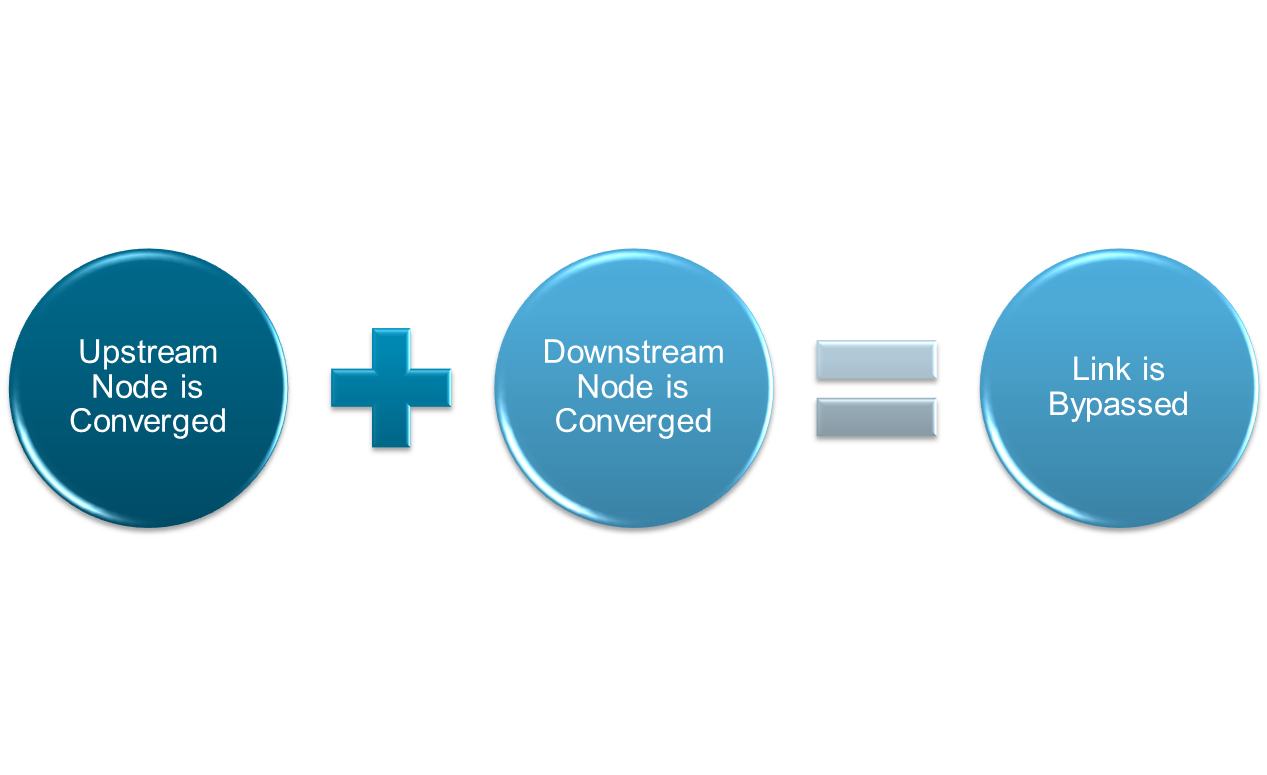## Wednesday, December 31, 2014

### What does Percent Not Converging Mean in SWMM5?

In SWMM 5 there is a Routing time step summary which tells the modeler the minimum time step during the simulation based on the CFL condition, the average time step, maximum time step, the percent of time spent in steady state, the average iterations per step and the percent not converging.  The percent not converging means the percent of the time the link flows and node depths did not converge within the maximum number of trials per time step (Figure 1).

*************************
Routing Time Step Summary
*************************
Minimum Time Step           :     0.52 sec
Average Time Step           :     8.76 sec
Maximum Time Step           :    10.00 sec
Percent in Steady State     :     0.00
Average Iterations per Step :     2.14
Percent Not Converging      :     1.69Figure 1.  Maximum number of trials per time step
The Percent Non Converging is the percent of time at least ONE link did not converge within the maximum number of trials per time step.  The Percent Non Converging is the Total Number of Non Convergent Steps divided by the total number of steps during the simulation.

Here is how the converging and non converging is determined in the dynamic wave solution.

The program keeps iterating until the Steps taken during this time step equals the Number of Maximum Trials. During each time step the link flows and the node depths are calculated.  If the Steps are greater than 1 and all nodes depths are converged (the difference between successive iterations is less than the Head tolerance) then the iteration process stops.  If even one node does not converge the Non Converge Count increases by one.   The links that can be bypassed during the next step are also estimated at each iteration. If a link can be bypassed the dynamic wave calculations are not performed and the program runs faster (Figure 2).Figure 2.  Link Bypass is based on the Upstream and Downstream Nodes

// --- keep iterating until convergence
while ( Steps < MaxTrials )
{
// --- execute a routing step & check for nodal convergence
initNodeStates();
converged = findNodeDepths(tStep);
Steps++;
if ( Steps > 1 )
{
if ( converged ) break;

// --- check if link calculations can be skipped in next step
}
}
if ( !converged ) NonConvergeCount++;

//  --- identify any capacity-limited conduits